# Online Electronics and Communication Engineering Test - Networks Analysis and Synthesis Test 1Loading Test...

Instruction:

• This is a FREE online test. DO NOT pay money to anyone to attend this test.
• Total number of questions : 20.
• Time alloted : 30 minutes.
• Each question carry 1 mark, no negative marks.
• DO NOT refresh the page.
• All the best :-).

1.

In an R-C series circuit excited by a voltage E, the charge across capacitor at t = 0+ is

A.
 0B.
 CEC.
 CE (1 - e-t/RC)D.
 none of the aboveExplanation:

Voltage across a capacitor cannot change instantaneously.

2.

In an unloaded transformer, the fluxes limiting the primary and secondary are 30 mWb and 20 mWb. The coefficient of coupling is

A.
 1B.
 0.1C.
 0.33D.
 0.67Explanation:

Φ11 = (1 - k) Φ1φ11 = φ1 - φ230 - 2010 mWb10 mWb = (1 - k) 30m0.33 = 1 - kk = 0.67 .

3.

In following circuit, steady state is reached with S open, S is closed at t = 0, the current I at t = 0+ is given by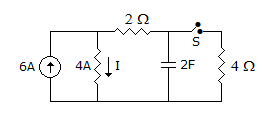A.
 9 ΩB.
 6 ΩC.
 7 ΩD.
 10 ΩExplanation:

When steady state is reached with s open, capacitor will act as open circuit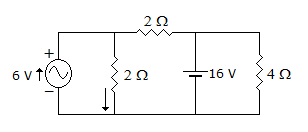Current in 4Ω resistor will be = 6A at steady state.

Voltage across 4Ω = 6 x 424 volt.

Current in 2Ω resistor due to 6A3A current due to 16 V, source.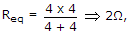I = 16/28A, this current divide in (2 + 2) Ω is 4Ω resistor current in 2Ω resistor4A + 3A7A.

4.

When a function Z(s) having a pole at infinity is realized, we get

A.
 a capacitance in seriesB.
 a resistance in seriesC.
 an inductance in seriesD.
 a resistance or inductance in seriesExplanation:

If we divide numerator by denominator we get term sL.

s Therefore the network has inductance in series.

5.

In the circuit shown in figure the inductor current and capacitor voltage are given at a time t. At this instant i is __________ A and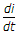is __________ A/sec.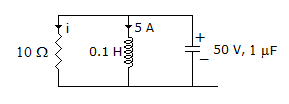A.
 -5 A, 10 VB.
 5 A, 50 VC.
 2.5, 25D.
 0, ∞Explanation: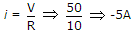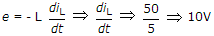.

6.

For an RC admittance function Y, the slope dY/dσ

A.
 is positiveB.
 is negativeC.
 may be positive or negativeD.
 07.

A network has only independent current sources and resistance. If values of all resistors are doubled, the values of node voltages

A.
 will become halfB.
 will remain unchangedC.
 will become doubleD.
 cannot be determined unless circuit configuration and values of resistors are known8.

Which of the following is/are correct? The circuit shown in the figure.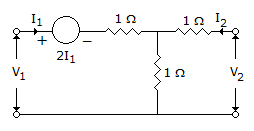1. is reciprocal
2. has Z11 = 2, Z22 = 2
3. has Z11 = 4, Z22 = 2
4. has Z11 = 0, Z22 = 2
Select the correct answer using the code given below:

A.
 1 and 3B.
 1 and 2C.
 1 and 4D.
 3 only9.

Force = Electric intensity x current.

A.
 TrueB.
 False10.

Which is correct for a transfer function?

A.
 The poles and zero must be complexB.
 If the poles and zeros are complex or imaginary, they must be conjugateC.
 If the poles and zeros are complex they must be simpleD.
 If a zero is imaginary, it must be simple11.

Which one of the following functions is RC driving point impedance?

A.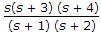B.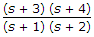C.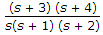D.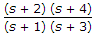12.

Consider the following statements regarding driving point admittance function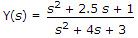1. It is an admittance of R1 network
2. Poles and zeros alternate on the negative real axis of plane
3. The lowest critical frequency is a pole
4. Y(0) = 1/3
Which of these statements are correct?

A.
 1, 2 and 3B.
 2 and 4C.
 1 and 3D.
 1, 2, 3 and 413.

The two port networks are connected in cascade, the combination is to be represented as a single two-Port network. The parameters of the network are obtained by multiplying the individual matrix

A.
 z-parameterB.
 h-parameterC.
 y-parameterD.
 ABCD parameter14.

At very low temperatures, semiconducting materials behave as insulators.

A.
 TrueB.
 False15.

A nickel cadmium cell is an alkaline cell.

A.
 TrueB.
 False16.

Current in a 2H inductance in 10e-t A. Admittance of the inductor is

A.
 -0.5 mhoB.
 0.5 mhoC.
 5 mhoD.
 10 mho17.

The flux linkage ψ, self inductance L and current i are related as

A.
 ψ = L/iB.
 ψ = LiC.
 ψ = Li2D.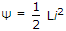18.

In liquids charge carriers are electrons only.

A.
 TrueB.
 False19.

For a transfer function H(s) = P(s)/Q(s), where P(s) and Q(s) are polynomials in s

A.
 the degrees of P(s) is always greater than degree of Q(s)B.
 the degree of P(s) and Q(s) are equalC.
 the degree of P(s) is independent of degree of Q(s)D.
 the maximum difference in degrees of P(s) and Q(s) is 120.

A function f(t) = k eat is __________ for (a > 0).

A.
 stableB.
 unstableC.
 marginalD.
 none